Etc. Created Edited

## Function

• Generate wave load parameters for static/dynamic analysis.

## Call

From main menu, select [Load] tab > [Type : Settlement/Etc.] > [Misc.] group >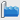[Wave Loads]

## Input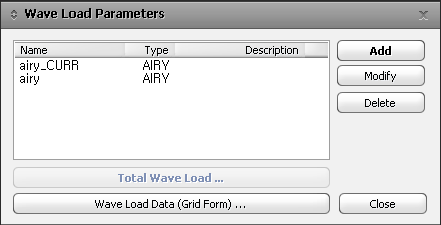Wave load parameter can be added, modified or deleted by clicking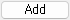,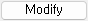,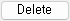Display the defined wave load in graph format.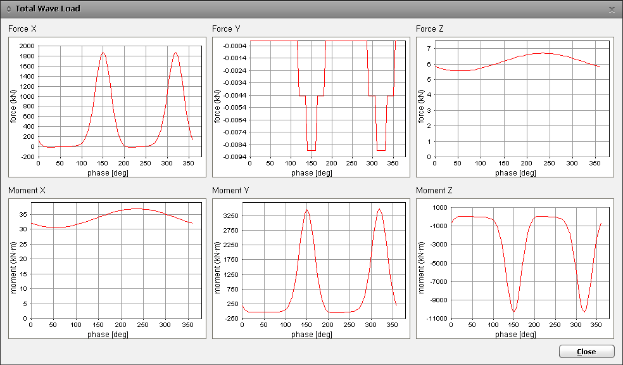Total Load Parameter List dialog box

### Wave Load Data (Grid Form)...

Display the defined wave load in the table format.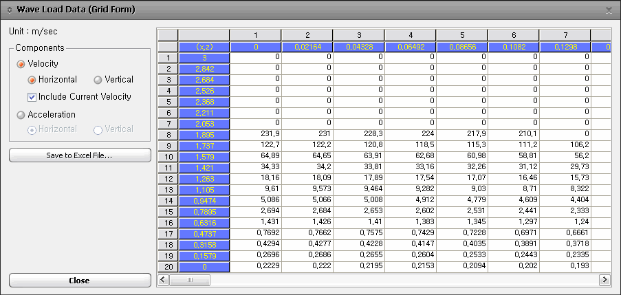Wave Load Data(Grid Form) dialog box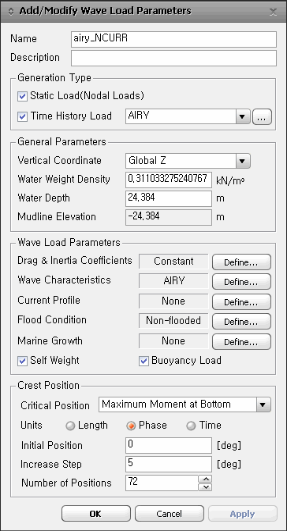### Generation Type

Generate wave loads based on the input wave information.

In order to convert into time history loads, Time History Load Case must be defined in advance. Click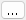button to generate Time History Load Case.

### General Parameters

Vertical Coordinate : Select the global axis of vertical direction of the wave load. Horizontal direction is always applied as Global X-axis.

Water Weight Density

Water Depth : Water depth based on the mean sea level (M.S.L)

Mudline Elevation : Vertical level of mud line with respect to the mean sea level (M.S.L)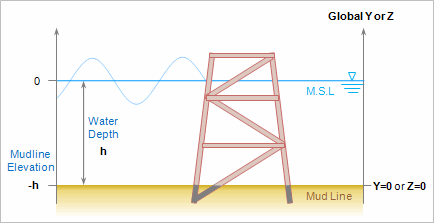위 그림에서 왼쪽 좌표는 해수면을 기준으로 표시한 것이고, 오른쪽 좌표는 모델링에서 사용할 수직좌표를 기준으로 표시한 것입니다.

Vertical Coordinate 항목에서 Global Z 좌표를 선택했다면, "Z=0"인 평면이 Mud line이 됩니다.

Z좌표가 (-)인 곳에 위치하는 요소는 퇴적층 아래에 위치하므로 파랑하중을 받지 않습니다. 즉, 위 그림의 구조물에서 진회색으로 표시한 부분에는 하중이 입력되지 않습니다.

1. Drag & Inertia Coefficients

You can enter the form coefficients to calculate the drag force (Cd) and the inertia force (Cm).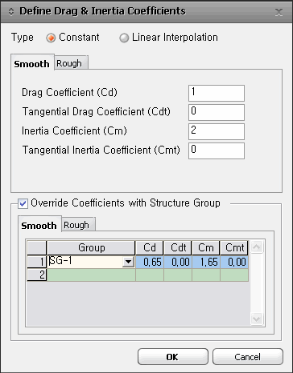Type

Constant : Apply the identical drag coefficient regardless of section diameter.

Linear Interpolation : Apply the drag coefficient by section diameters. The program performs linear interpolation for the diameters not specified in the table.

Drag & Inertia Coefficient

Diameter : The projected diameter of the member in the wave direction

Drag Coefficient Cd : Drag coefficient applied to the perpendicular direction of an element

Tangential Drag Coefficient Cdt : Drag coefficient applied to the tangential direction of an element

Inertia Coefficient Cm : Inertia coefficient applied to the perpendicular direction of an element

Tangential Inertia Coefficient Cmt : Inertia coefficient applied to the tangential direction of an element

Override Coefficients with Structure Group

Enter the drag and inertia coefficient by structure group.

2. Water Characteristics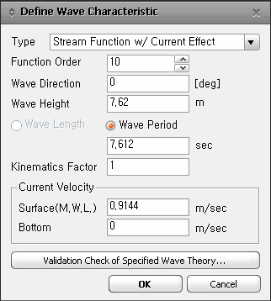Type : The available wave theories for consideration are as follows:

Airy Wave

Airy Wave (mean position)

Stokes V Wave

Stream Function w/ Current Effect

Stream Function w/o Current Effect

Cnoidal Wave

Solitary Wave

Function Order : Order of Stokes wave theory

Wave Direction : Wave load direction. Enter the degree from Global X-axis in counterclockwise direction.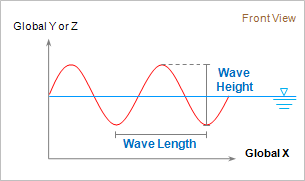Wave Height

Wave Length

Wave Period : Wave period and wave velocity through one wavelength.

Kinematics Factor : Factor considering irregular behavior of wave load. 0 ＜ Kinematics factor ≤ 1

Current Velocity

Surface(M.W.L) : Velocity of current at the mean water level (M.W.L)

Bottom : Velocity of current at the bottom of sea

Validation Check of Specifide Wave Theory : Validate the specified wave load data based on the wave theory.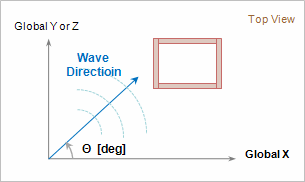3. Current Profile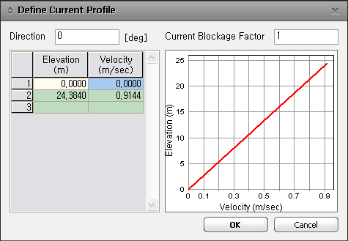Current loads will be generated by entering data in the Current Profile and will be included in the wave load cases. Note that these data will be neglected when "Stream Function with Current Effect" type is selected for the wave load type.

Direction : Refer to the figure below for wave direction.

Current Blockage Factor : Blockage factor of current due to structure

Elevation : 0 ≤ Elevation ≤ Depth of water(h)

Velocity : Velocity of current

4. Flood Condition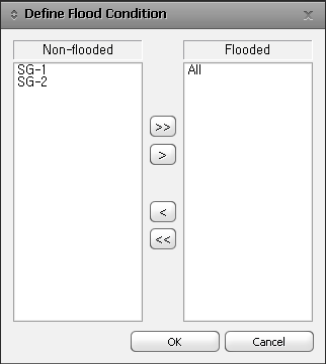Select the member that is filled with seawater inside the cross-section. Pre-assign the corresponding member to a group and choose the group that represents the Flooded Member.

5. Marine Growth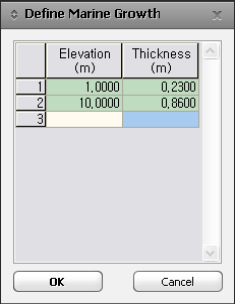Consider the variation in thickness of the structure due to marine growth.

### Crest Position

Enter the Critical Position and Step for Wave Load calculation.

Critical Position : Generate nodal loads which will cause one of the following items depending on the position of crest.

Maximum Moment at Bottom

Minimum Moment at Bottom

Maximum Shear at Bottom

Minimum Shear at Bottom

Maximum Force Upward

Maximum Force Downward

Initial Position : Initial position/degree/time of wave load

Increase Step : Increment of crest position

Number of Positions : Number of positions to be calculated

0